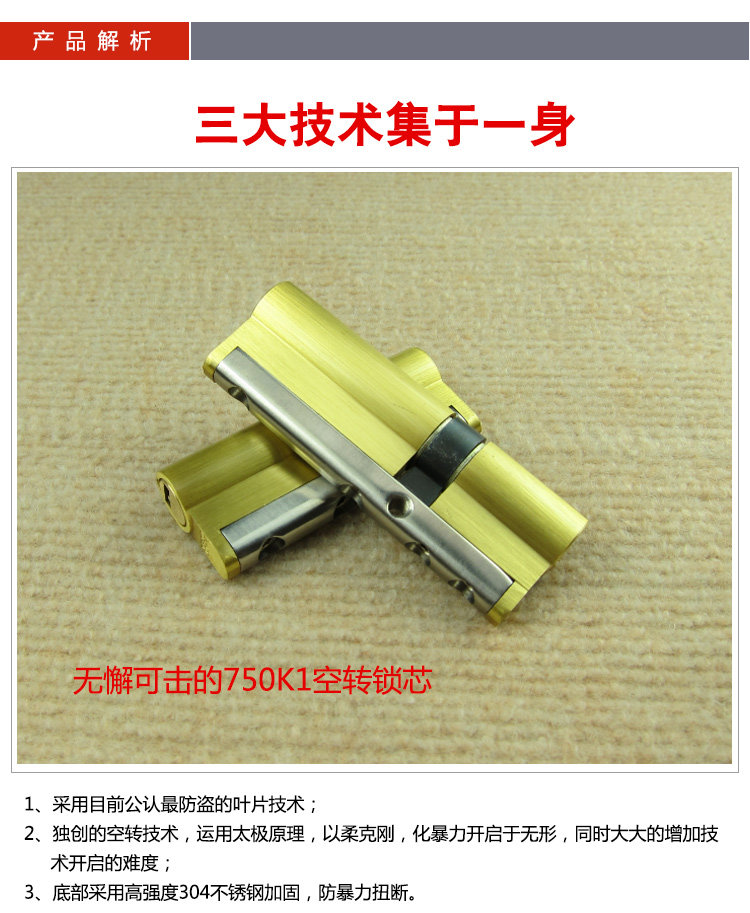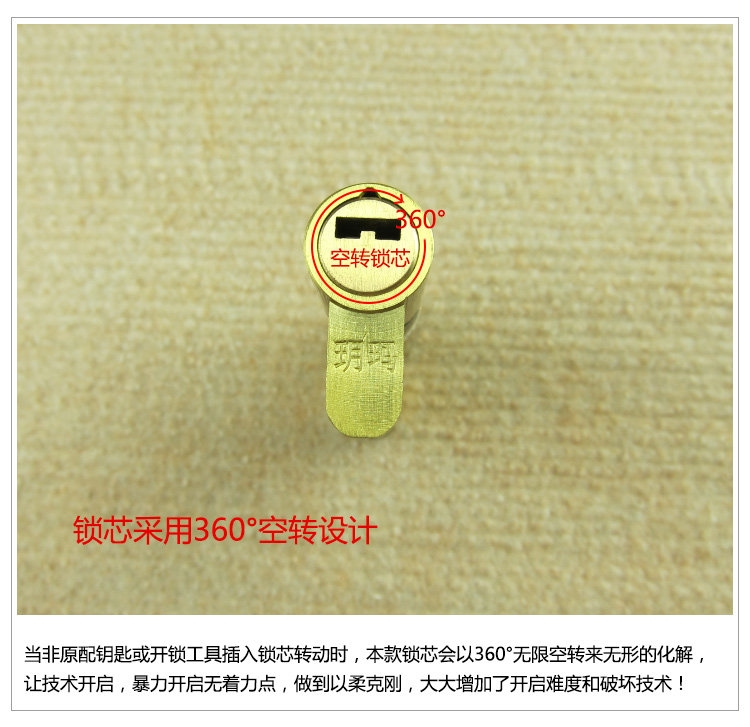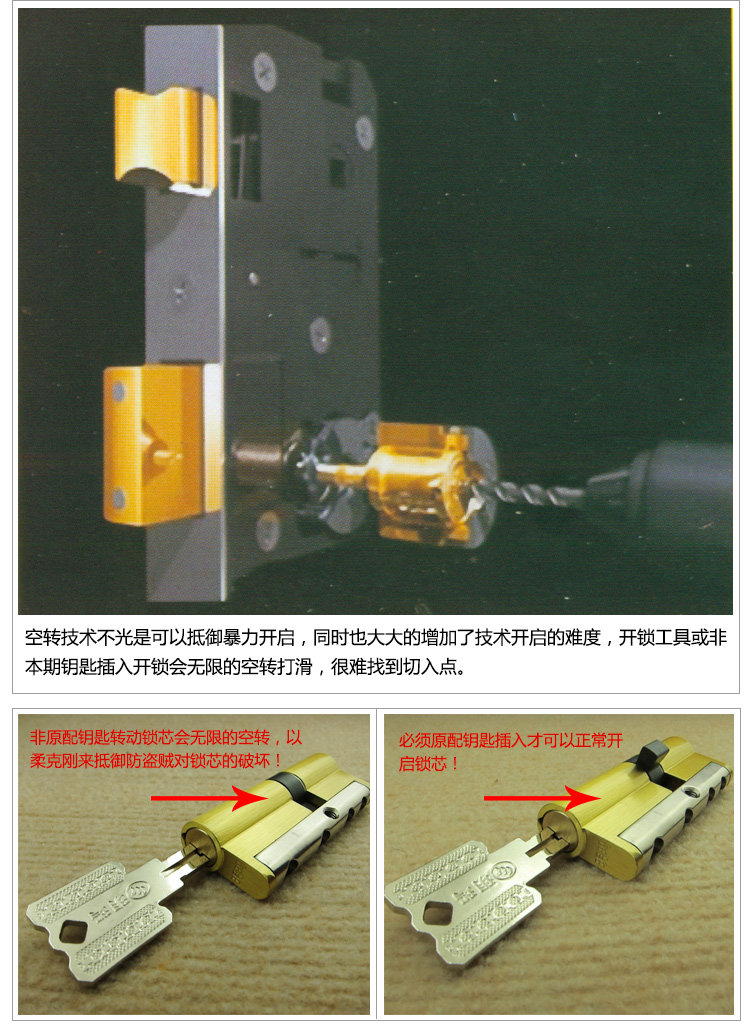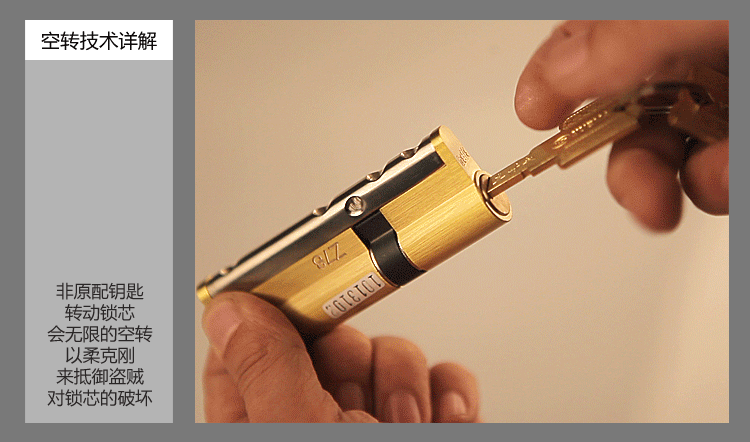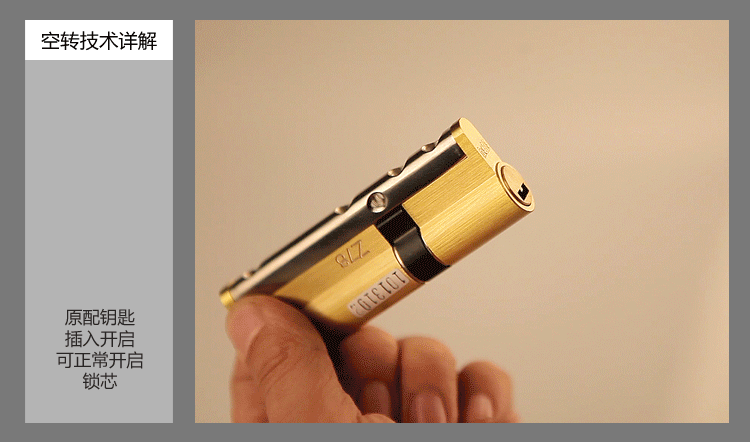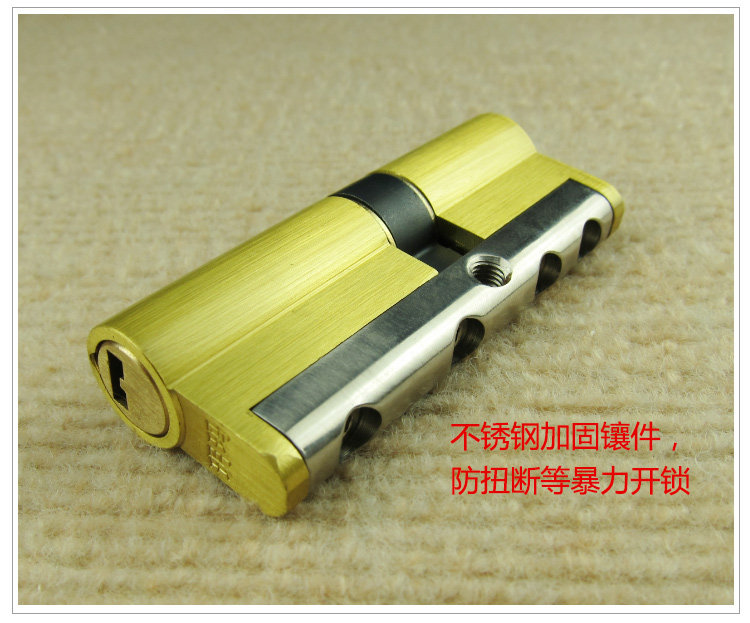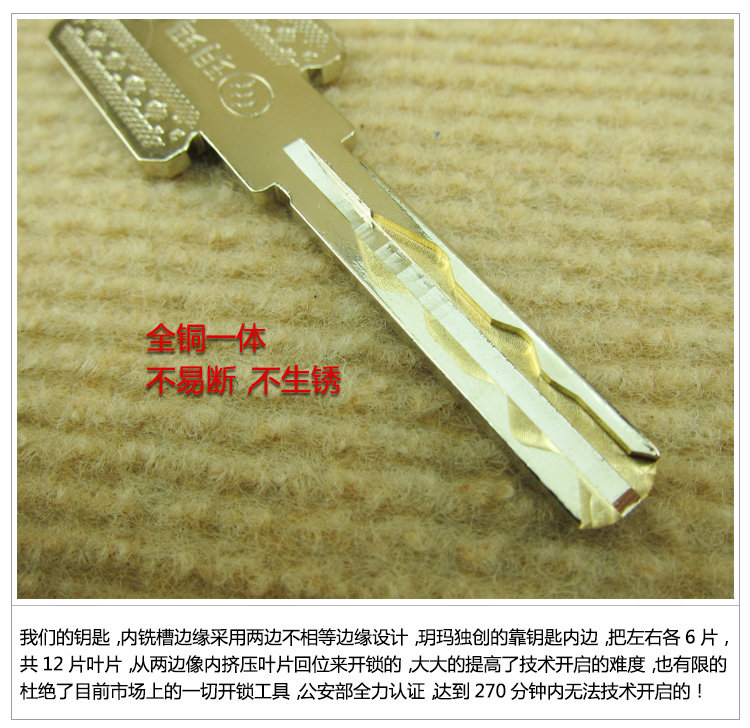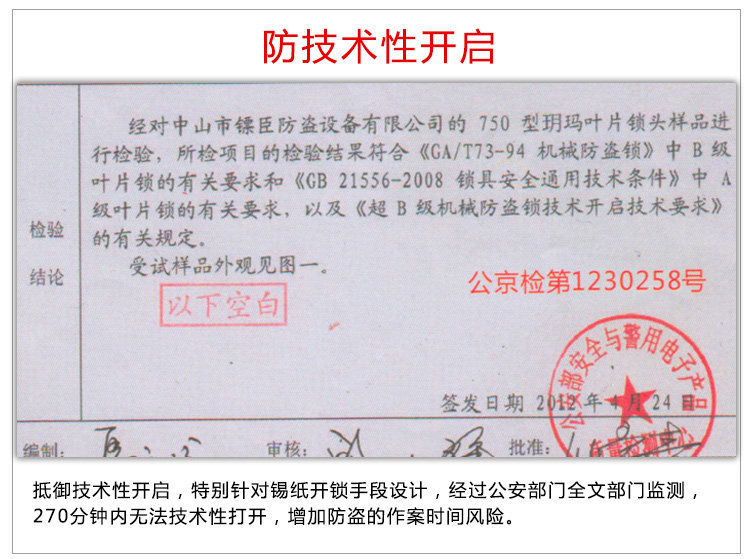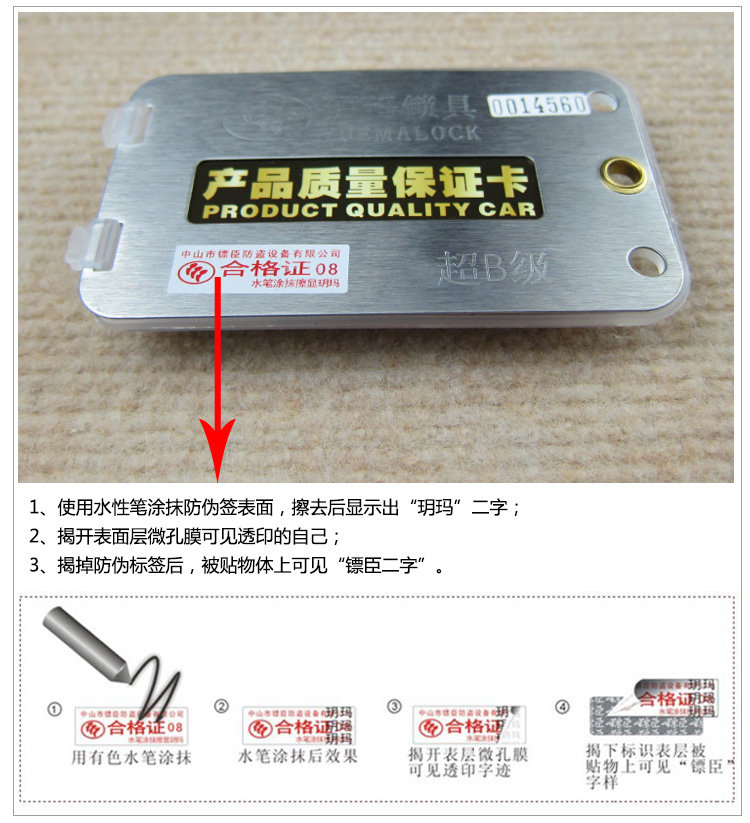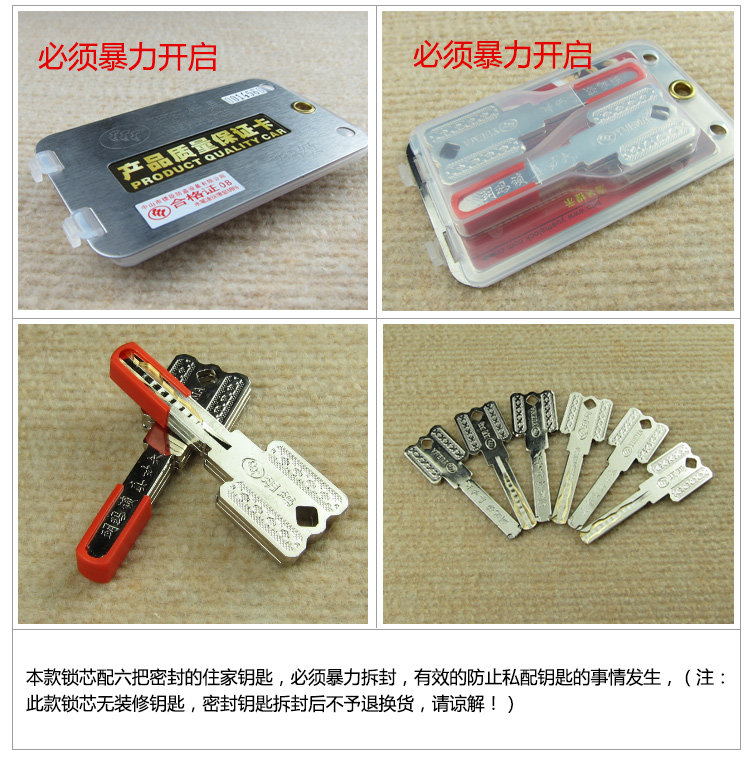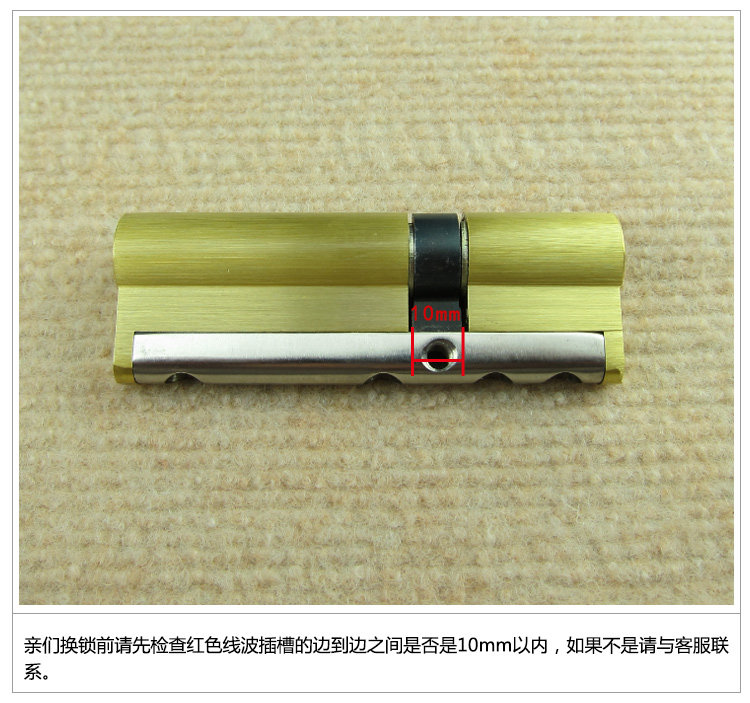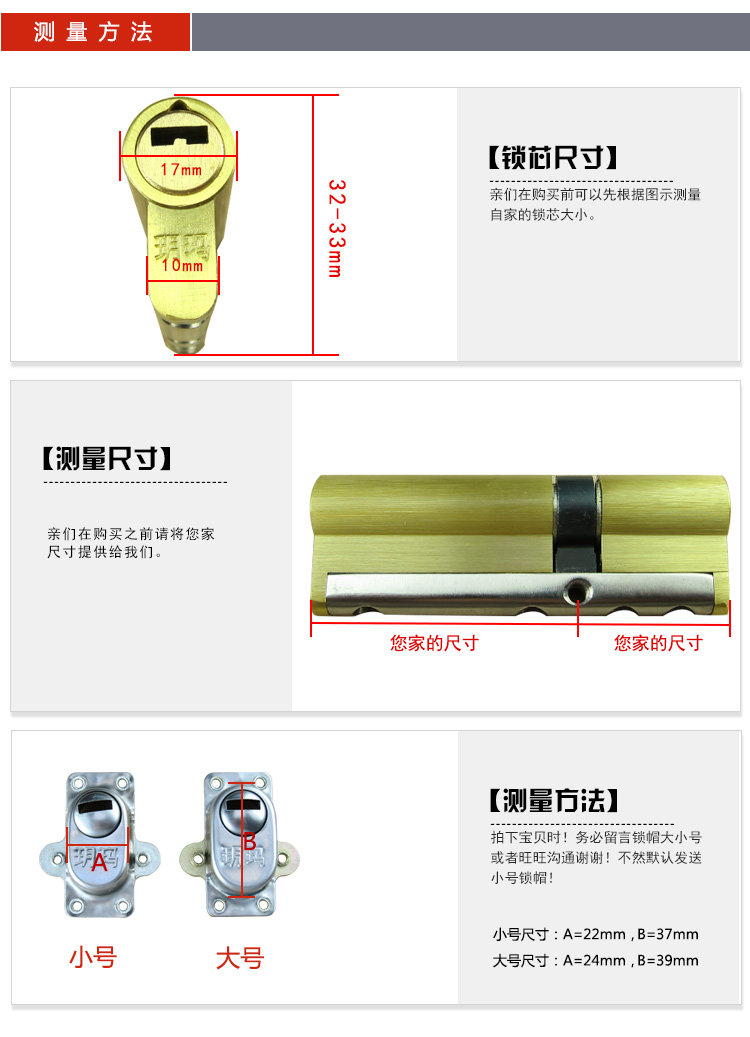QQ：13357357963

# 玥玛750K1空转叶片超B级锁芯

• 品牌: 玥玛
• 型号: 750-K1
• 把手材质: 铜
• 锁舌材质: 铜
• 锁芯材质: 铜
• 适配门厚度: >55mm
• 功能用途: 锁大门
• 中心距: 其他
• 锁边距: 其他
• 面板材质: 其他
• 饰面颜色: 32.5+32.5=65中K1 35+35=70中K1 30+40=70偏K1 37.5+37.5=75中K1 32.5+42.5=75偏K1 40+40=80中K1 32.5+47.5=80偏K1 42.5+42.5=85 中K 32.5+52.5=85偏K1 45+45=90中K1 32.5+57.5=90偏K1 32.5+62.5=95偏K1 47.5+47.5=95中K1 请留言锁帽大小号（不留默认小号 其他尺寸请咨询店主
• 弹珠材质: 不锈钢叶片结构
• 锁舌类型: 其他
• 开门方向: 通用型
• 是否带钥匙: 带钥匙